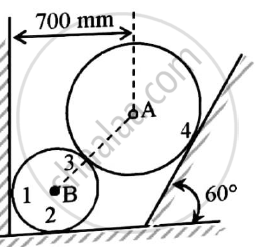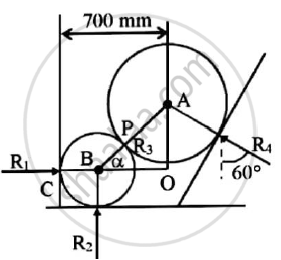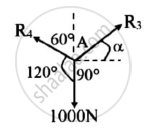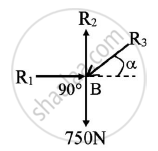# Two Spheres a and B of Weight 1000n and 750n Respectively Are Kept as Shown in the Figure..Determine Reaction at All Contact Points 1,2,3 and 4. Radius of a is 400 Mm and Radius of B is 300 Mm - Engineering Mechanics

Two spheres A and B of weight 1000N and 750N respectively are kept as shown in the figure..Determine reaction at all contact points 1,2,3 and 4. Radius of A is 400 mm and radius of B is 300 mmGiven  : Two spheres are in equilibrium
W1=1000 N
W2=750 N
rA=400 mm
rB=300 mm
To find : Reaction forces at contact points 1,2,3 and 4

#### Solution

BC = BP = 300mm = 0.3m
AP = 400 mm = 0.4 m
AB = AP + BP
= 0.7m
CO = BC + BO
0.7 = 0.3 + BO
BO = 0.4m

In △AOB
cos α = (BO)/(AB) = (0.4)/(0.7)
α = cos-1(0.5714)
α = 55.1501Forces R3,R4 and 1000N are under equilibrium at point AApplying Lami’s theorem
(R3)/(sin120) = 1000/(sin (150−alpha)) = (R4)/(sin (90+alpha))

(R3)/(sin120) = 1000/(sin (150−55.1501)) = (R4)/(sin(90+55.1501))
Solving the equations
R3 = 869.1373 N
R4 = 573.4819 NForces R1,R2,R3 and 750N are under equilibrium at B
Applying conditions of equilibrium

ΣFY=0
-R3sin α-750+R2=0
R2=869.1373 sin55.1501+750
R2=1463.2591 N (Acting upwards)
Applying conditions of equilibrium

ΣFX=0
R1-R3cosα=0
R1=869.1373cos55.1501

R1=496.65 N(Acting towards right)

 Sr.no. Point Force 1. R1 496.65 N(Towards right) 2. R2 1463.2591 N(Towards up) 3. R3 869.1373 N(55.1501o in first quadrant) 4. R4 573.4819 N(30o in second quadrant)
Concept: Condition of Equilibrium for non-concurrent nonparallel general forces
Is there an error in this question or solution?